×#### Thank you for registering.

One of our academic counsellors will contact you within 1 working day.

Click to Chat

1800-1023-196

+91-120-4616500

CART 0

• 0

MY CART (5)

Use Coupon: CART20 and get 20% off on all online Study Material

ITEM
DETAILS
MRP
DISCOUNT
FINAL PRICE
Total Price: Rs.

There are no items in this cart.
Continue Shopping• Complete JEE Main/Advanced Course and Test Series
• OFFERED PRICE: Rs. 15,900
• View Details

Chapter 1: Rational Numbers Exercise – 1.2

Question: 1

Verify commutativity of addition of rational numbers for each of the following pairs of rational numbers.Solution:

Commutativity of the addition of rational numbers means that if ab and cd are two rational numbers, then ab + cd = cd + ab.

(i) We have:Hence, verified.

(ii)  We have: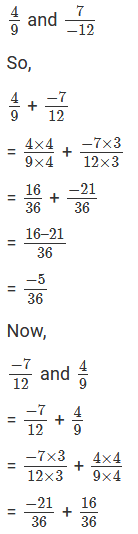Hence, Verified.

(iii)  We have:Hence, verified.

(iv) We have:Hence, verified.

(v) We have:Hence, verified.

(vi) We have: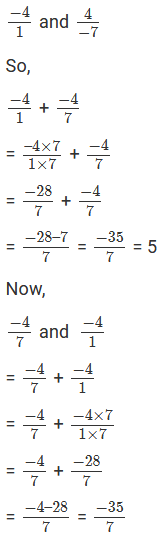Hence, verified.

Question: 2

Verify associativity of addition of the rational numbers i.e.,

(x + y) + z = x + (y + z), when: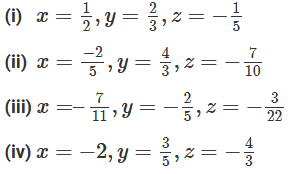Solution:

We have to verify that: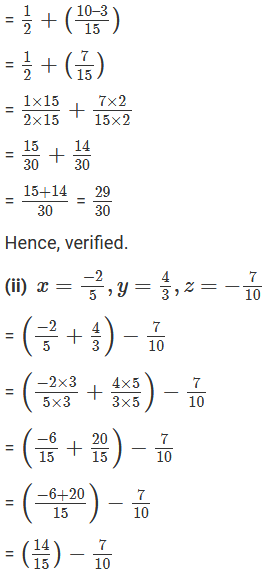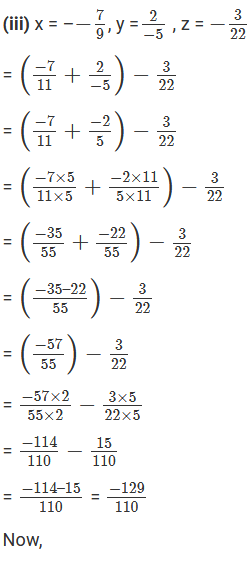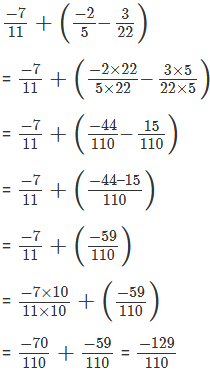Hence, verified.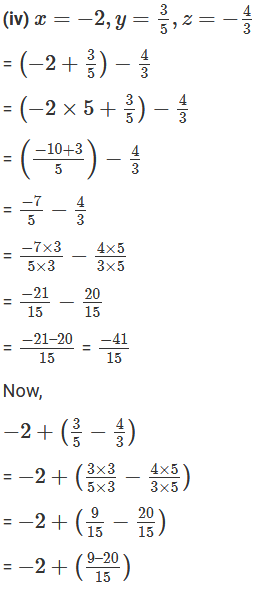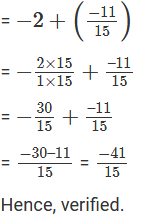Question: 3

Write the additive inverse of each of the following rational numbers: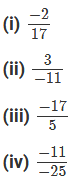Solution:

(i) Additive inverse is the negative of the given number.

So, additive inverse of -2/17 = 2/17

(ii) Additive inverse is the negative of the given number.

So, additive inverse of 3/-11 = 3/11

(iii) Additive inverse is the negative of the given number.

So, additive inverse of -17/5 = 17/5

(iv) Additive inverse is the negative of the given number.

So, additive inverse of -11/-25 = 11/25

Question: 4

Write the negative (additive inverse) of each of the following:

(i) -25

(ii) 7-9

(iii) -1613

(iv) -51

(v) 0

(vi) 1

(vii) -1

Solution:

(i) Additive inverse of −2/5 = 2/5

(ii) Additive inverse of −7/9 = 7/9

(iii) Additive inverse of −16/13 = 16/13

(iv) Additive inverse of −5/1 = 5/1

(v) Negative value of 0 is 0

(vi) Negative value of 1 is -1

(vii) Negative value of -1 is 1

Question: 5

Using commutativity and associativity of addition of rational numbers, express each of the following as a rational number: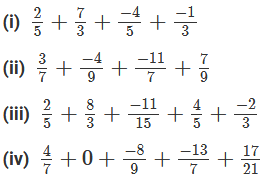Solution:

(i)  We have: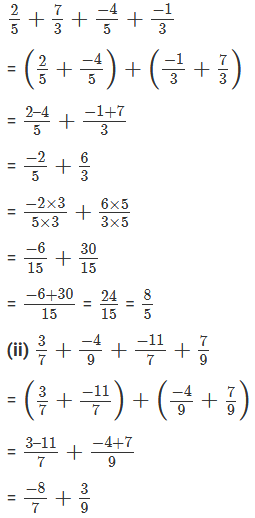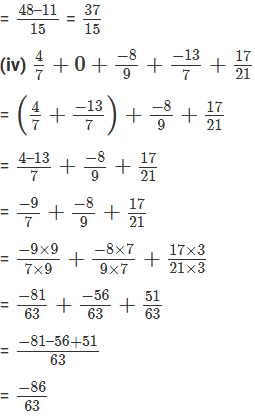Question: 6

Using commutativity and associativity of addition of rational numbers, express each of the following as a rational number: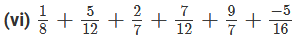Solution: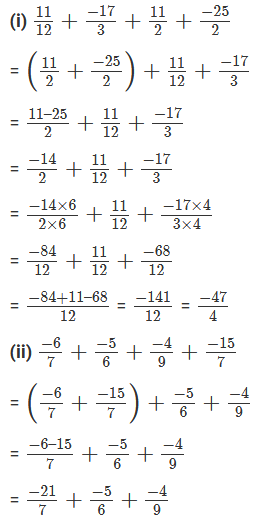### Course Features

• 728 Video Lectures
• Revision Notes
• Previous Year Papers
• Mind Map
• Study Planner
• NCERT Solutions
• Discussion Forum
• Test paper with Video Solution